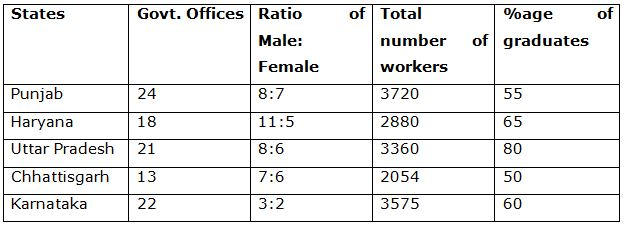# LIC HFL Associate/ Assistant Numerical Ability Questions – (Day-05)

Dear Aspirants, Our IBPS Guide team is providing new series of Numerical Ability Questions for LIC HFL Associate/ Assistant 2019 so the aspirants can practice it on a daily basis. These questions are framed by our skilled experts after understanding your needs thoroughly. Aspirants can practice these new series questions daily to familiarize with the exact exam pattern and make your preparation effective.

Check here for LIC HFL Mock Test 2019

Check here for IBPS RRB PO Mains Mock Test 2019

Check here for IBPS RRB Clerk Mains Mock Test 2019

### Click Here to Subscribe Crack High Level Puzzles & Seating Arrangement Questions PDF 2019 Plan

[WpProQuiz 7044]

Directions (1 – 5): What approximate value should come in the place of question mark (?) in the following questions?

1) 47% of 1898 + 31% of 4502 =? × 27 + 1452 + 913.89 – 293.89

a) 14

b) 20

c) 8

d) 12

e) 26

2) 6 1/5 of 19475 + 7 2/7 of 308.29 =? % of 604 + 858.85

a) 20355

b) 18750

c) 16425

d) 8920

e) 23575

3) 516 × 3719 ÷ 31 % of 2399 = 64 × 125.89 ÷ 7.21 + ? – 1899

a) 4170

b) 4950

c) 3890

d) 3330

e) 5160

4) 514 × 6298. 81 ÷ 24 % of 749 = 18 × 439.71 ÷ 11.12 + ? + 2599

a) 18920

b) 14670

c) 21560

d) 23780

e) 26790

5) (4/11) of 176.18 × 45.12 ÷ 14.78 – ? = 855.31 ÷ 9 – 434.98

a) 780

b) 600

c) 700

d) 660

e) 530

Directions (6 – 10): Study the table carefully and answer the following questions.6) The number of male graduates in Uttar Pradesh is 1800. If the number of female graduate increase by 50% in the next year, approximately what percent of female workers in that particular state is graduate?

a) 93%

b) 95%

c) 87%

d) 125%

e) 75%

7) What is the ratio between the total number of male workers in states Haryana and Karnataka to the total number of graduate workers in the same states?

a) 1:2

b) 1375:1339

c) 2:5

d) 841:902

e) None of these

8) What is the total number of graduate workers from all the states?

a) 9778

b) 9017

c) 9885

d) 9025

e) None of these

9) Which state has the lowest number of average workers per office?

b) Karnataka

c) Haryana

d) Punjab

e) None of these

10) In which state percentage of female workers to number of workers (both male and female) is ranked third highest?

a) Chhattisgarh

b) Haryana

c) Punjab

e) None of these

Direction (1-5) :

47% of 1900 + 31% of 4500 = 27x + 1452 + 914 – 294

(47/100)*1900 + (31/100)*4500 = 27x + 1452 + 914 – 294

893 + 1395 – 1452 – 914 + 294 = 27x

27x = 216

X = 8

(31/5)*19475 + (51/7)*308 = (x/100)*600 + 859

120745 + 2244 = 6x + 859

120745 + 2244 – 859 = 6x

6x = 122130

X = 20355

516 × 3720 ÷ 31 % of 2400 = 64 × 126 ÷ 7 + x – 1900

516 × 3720 ÷ [(31/100)*2400] = 64*(126/7) + x – 1900

516 × 3720 ÷ 744 = 1152 + x – 1900

516*(3720/744) = 1152 + x – 1900

2580 – 1152 + 1900 = x

X = 3328 = 3330

514 × 6300 ÷ (24/100)*750 = 18*(440/11) + x + 2600

514*6300/180 = 720 + x + 2600

17990 -720 – 2600 = x

X = 14670

(4/11)*176*(45/15) – x = (855/9) – 435

192 – x = 95 – 435

192 – 95 + 435 = x

X = 532 = 530

Direction (6-10) :

= (3360*80)/100 = 2688

Number of female graduates = 2688 – 1800 = 888

Females increases by 50% = (888*150)/100 = 1332

Total number of female in Uttar Pradesh = (6*3360)/14 = 1440

Required % = (1332*100)/1440 = 92.5% = 93% (approx.)

Required ratio

= [(11/16)*2880 + (3/5)*3575]/[(65/100)*2880 + (60/100)*3575]

= (1980+2145)/(1872+2145) = 4125/4017

= 1375:1339

Total number of graduate workers from all the states

= 3720*(55/100) + 2880*(65/100) + 3360*(80/100) + 2054*(50/100) + 3575*(60/100)

= (2046+1872+2688+1027+2145) = 9778

Punjab = 3720//24 = 155

Haryana = 2880/18 = 160

Uttar Pradesh = 3360/21 = 160

Chhattisgarh = 2054/13 = 158

Karnataka = 3575/22 =162

Hence, Punjab has the lowest number of average workers.

Punjab = 7/15*100 = 46.67%

Haryana = 5/16*100 = 31.25%

Uttar Pradesh = 6/14*100 = 42.86%

Chhattisgarh = 6/13*100 = 46.15%

Karnataka = 2/5*100 = 40%